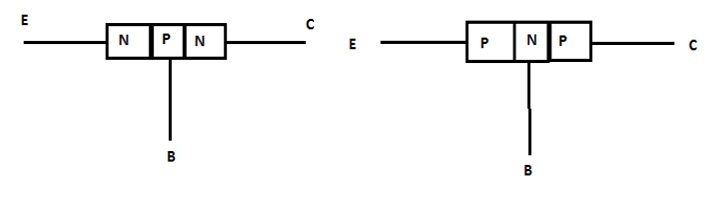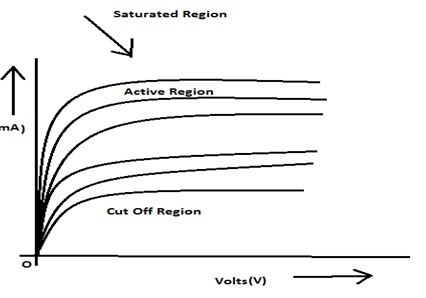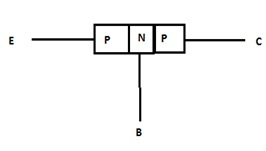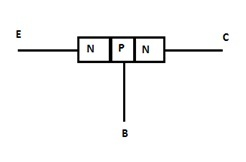# Bipolar Junction Transistor: Definitions, Construction, Types

## Introduction

Bipolar junction transistor is a semiconducting device. Silicon or Germanium are the two semiconductors used in Bipolar junction transistors. Three layers formed in between the two P-N junctions. They are named emitter, base, and collector.• Emitter layer represented as E

• Collector layer represented as C

• Base layer represented as B

The middle part of the transistor is known as Base layer (B). The base layer is the thin layer. The thickness of the base layer is 25 microns. The left side layer of the transistor is the Emitter layer (E). The right-side layer of the transistor is known as the Collector layer(C).

## Bipolar Junction Transistors

A device used to amplify a signal consisting of a three-layered semiconductor material also with a two P-N junction is called Bipolar Junction Transistors (BJT). When the external power supply is applied, the transistor is ready to be amplified. The base layer is the thin layer. The left side part of the transistor is the Emitter layer (E). The right side part of the transistor is known as the Collector layer(C). The emitter layer is highly doped.

But the emitter has to provide most charge carriers. The base layer is opposite to the emitter because it is faintly doped. The Collector layer is also faintly doped to receive more transporters. From these characteristics, we should know collector and emitter cannot be exchanged.## Bipolar Junction Transistor symbol

The arrowhead always represents the current flow direction emitter region to base or base to emitter (from P to N) in every transistor. It denotes the conventional current flow direction. In the PNP transistor, the arrowhead faces the base region. It shows that emitted is positive for base and collector. In an NPN transistor, the arrowhead faces the emitter region. It means the base is positive for the emitter region.

## Bipolar Junction Transistor construction

It is made up of a separate piece of the semiconductor element with two consecutive P-N junctions. Silicon or Germanium are the two semiconductors used in Bipolar junction transistors. We already know transistors consist of three layers: emitter, base, and collector. In the PNP transistor, two PN junctions are there. The left side is connected to the emitter. The middle part is the base region and the right side is a collector layer. E-B and C-B intersection are the two junctions of the BJT. E-B intersection are forward biased and C-B intersection are reverse biased.

## Operation of Bipolar Junction Transistor

Operating regions of BJT are the saturated region, the cut-off region, and the active region.

### Saturation Region

The starting point of the turn is called the saturation region. The Saturation area is in the middle of the origin and constant point where the collector current becomes constant.### Cut Off Region

Even base current is zero but a very low collector current is present in the transistor. The low region, where the base current is zero, that region is known as the Cut-off Region.

### Active Region

The active area is placed in the middle of the curve. It is centered between the saturated and cut-off regions. Turns in the active region are constant. In this area, the emitter-base terminal is forward biased and the collector-base terminal is reverse biased.

• PNP BJT

• NPN BJT

## PNP BJTIn PNP BJT, both side of N-type material are filled with P-type semiconductors.

## NPN BJTIn an NPN BJT, the left side and right side are filled with n-type semiconductors. The p-type semiconductor is placed between these two semiconductors. When current enters the emitter region it converts the positive sign into a negative sign.

## Function Of Bipolar Junction Transistor

PNP BJT and NPN BJT are the two types of bipolar junction transistors. There is a back to back PN junctions present in the PNP transistors. Potential barriers are increased because of the depletion layer present in these two junctions. The batteries supply external power. Because of the forward biasing lots of holes pass the terminal and enter the base. Some electrons are enter into emitter from the base. These electrons reunite with holes in the emitter. Emitter current IE increases when the drift of electrons increses from the emitter to the base. Likewise, Base current and, Collector current flow was calculated. These is the functions of the Bipolar Junction Transistor.

## Voltage, charge control and, Current configuration of Bipolar Junction Transistors

The C-E current controls the B-E current. The collector-emitter current gives the relation between current-voltage. Concentrated secondary carriers are in the collector current because it has a base region.

To operate a Bipolar Junction Transistor there are three types of circuit connections.

• Common Base (CB) mode

• Common Emitter (CE) mode

• Common Collector (CC) mode.

## Applications of BJT

### Transistors As a Switch

Normally BJT is used as a switch. For example, consider a common emitter configuration of an NPN transistor. The Collector is linked in series with Load resistance and base resistance. Transistor is saturated when the given input is high. Maximum current will flow in the circuit when the transistor is saturated. So, the transistor is in the ON stage. When the given input is low, no current is flow in base-emitter junction. The transistor will be in the OFF stage.

### Transistor Amplifier

Amplification is the major function of Bipolar Junction Transistors.

## Conclusion

Transistor is a device used to step-up a signal consisting of three-layers. The semiconductor material with a two P-N junction is called Bipolar Junction Transistors (BJT). It consists of three layers; Emitter, Collector, and, Base. There are two types of BJT i.e. PNP BJT and NPN BJT. The main function of BJT is calculating the current flow of the three layers. Application of BJT is used as a switch, amplifiers, filters, and oscillators.

## FAQs

Q1. What is Transistor Biassing?

Ans. When a signal passes through a circuit, emitter voltage should be maintained and the selection of operating point must be perfect. This process is called Transistor biassing.

Q2. What is output impedance?

Ans. At a constant current base ratio the difference in C-E voltage to the parallel difference in collector current in the active region is known as Output impedance.

$$\mathrm{Output\:impedance,\:r_0=(\frac{\Delta V_{CE}}{\Delta I_C})I_B}$$

Q3. What are the three characteristics of transistors?

Ans.

• Input Characteristics

• Output Characteristics

• Transfer Characteristics

Q4. Mention the biasing conditions under which a transistor can work.

Ans.

• The emitter-base terminal is forward biased so that a lots of charge carriers are pushed back from the emitter and a small amount of resistance is provided by the junction.

• The collector-base terminal is reverse biased so that it accepts a high number of charge carriers and a high amount of resistance provided by the junction to the current.

Q5. Define current gain β

Ans. At a constant collector-emitter voltage, the ratio of a variation of the collector current to the parallel difference of the base current is called Current gain.

$$\mathrm{Current\:Gain\:β=(\frac{\Delta I_C}{\Delta I_B})_{V_{CE}}}$$

Updated on: 28-Apr-2023

742 Views